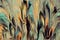Photo by Peter Olexa on Unsplash

# Dissecting 1-Way ANOVA and ANCOVA with Examples in R

## Differences in Means by Analyzing the Variance

ANOVA (Analysis of Variance) is a process to compare the means of more than two groups. It can also be used for comparing the means of two groups. But that’s unnecessary. Comparing the means between two groups only can be done using a hypothesis testing method such as a t-test.

If you need a refresher on the t-test or z-test please check this article:

This article will focus on comparing the means of more than two groups using the Analysis of Variance (ANOVA) method. This method breaks down the overall variability of a given continuous outcome into pieces.

## One-Way Analysis of Variance

One way…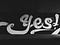Photo by Jon Tyson on Unsplash

# Complete Details of Simple Logistic Regression Model and Inference in R

## Demonstration with Example

One of the most basic, popular, and powerful statistical models is logistic regression. If you are familiar with linear regression, logistic regression is built upon linear regression. It uses the same linear formula just a bit different implementation. This article will discuss the details of logistic regression in R. But for a refresher or better understanding, I will discuss some formulas behind the model.

If you need a refresher on linear regression, please feel free to go through this article:

## Simple Logistic Regression

As I mentioned before this uses the same linear formula as linear regression. Then what is the difference between linear…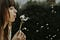Photo by nine koepfer on Unsplash

# The Ultimate (Free) Data Visualization Compilation for Python

## Learn to use more visualization functions and techniques

Data Visualization is essential if you deal with data in any way. I focus on that a lot. I wrote several articles before on data visualization in Python. I realized if I compile them on one page it may become a huge collection of data plotting techniques in one place. The amount of data visualization you may learn from here might rival any paid visualization course out there.

## Matplotlib

This is arguably the most popular and most used visualization library in Python. There are other high-quality libraries of python that are built on Matplotlib. Even if you use some other libraries…

Thank you so much for checking out my blog! Actually, readers may read even if it is not in a publication. When it is in a publication, that publication's followers see it in their feed. So the article gets more visibility.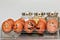Photo by Tengyart on Unsplash

# Take Full Control Over the Subplots in Matplotlib

## Use of Subplots and GridSpec Together for Better Control

Using subplots and putting multiple plots in one figure can be very useful in summarizing a lot of information in a small space. They are helpful in making reports or presentations. This article will focus on how to use subplots efficiently and take fine control over the grids.

We will start with the basic subplot function to make equal size plots first. Let’s do the necessary imports:

`%matplotlib inlineimport matplotlib.pyplot as plt import numpy as np import pandas as pd`

Here is the basic subplots function in Matplotlib that makes two rows and three columns of equal-sized rectangular space:

`…`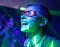Photo by Sarah Wolfe on Unsplash

# A Complete Guide to Confidence Interval, t-test, and z-test in R for Data Scientists

## Lots of Hands-on Exercises

The confidence interval, t-test, and z-test are very popular and widely used methods in inferential statistics. They are so important because, for any research or data analysis, we can only use a sample to come to a conclusion about a large population. In that case, these inferential statistical methods help us consider the errors and infer a better estimate for a larger population using a smaller sample.

You may think there is a lot to cover in one article. Yes, they are actually a lot to digest in one day. …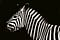Photo by redcharlie on Unsplash

# A Complete Logistic Regression Algorithm for Image Classification in Python From Scratch

## Detailed layout of a logistic regression algorithm with a project

Logistic regression is very popular in machine learning and statistics. It can work on both binary and multiclass classification very well. I wrote tutorials on both binary and multiclass classification with logistic regression before. This article will be focused on image classification with logistic regression.

If you are totally new to logistic regression, please go to this article first. This article has a detailed explanation of how a simple logistic regression algorithm works.

It will be helpful if you are familiar with logistic regression already. If not, I hope you will still understand the concepts here. …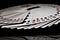Photo by Jarosław Kwoczała on Unsplash

# A Complete Overview of the Probability Distributions with Examples, R Implementation

## Most Popular and Widely Used Discrete and Continuous Probability Distributions

Our end goal is to draw inferences from the population. We first need to learn about probability because it is the underlying base for statistical inferences, predictive models, and machine learning algorithms.

Different types of probability distributions help to find the probability of the occurrence of an event. Different types of distribution work in different conditions.

In this article, I will discuss:

1. The basics of the probability distribution

2. The ideas and properties of different types of distributions

3. The formula and how to calculate different types of discrete distributions with examples.

4. Implementation of different types of distributions in…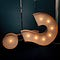Photo by Jon Tyson on Unsplash

# Do You Need A Masters Degree to Become a Data Scientist?

## My Own Experience and Realization Being a Student of MS in Data Analytics at Boston University

Given the hype going on about data science, this is a very valid question, do you need a master's degree. If this hype is genuine or not is also another big question. But this article will focus on if a master's degree is necessary. There are so many other short, easier, and cheaper options out there. In fact so many free courses in Coursera, edx, and learning sites. Is it still necessary to go through a big academic process? Assignments, quizzes, exams, term projects, presentations?

This article will cover:

1. A little bit about my own journey
2. How I feel about…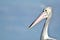Photo by Mark Stoop on Unsplash

# An Ultimate Cheat Sheet for Data Visualization in Pandas

## All the Basic Types of Visualization That Is Available in Pandas and Some Advanced Visualization That Are Extremely Useful and Time Savers

We use python’s pandas' library primarily for data manipulation in data analysis. But we can use Pandas for data visualization as well. You even do not need to import the Matplotlib library for that. Pandas itself can use Matplotlib in the backend and render the visualization for you. It makes it really easy to makes a plot using a DataFrame or a Series. Pandas use a higher-level API than Matplotlib. So, it can make plots using fewer lines of code.

I will start with the very basic plots using random data and then move to the more advanced one with…## Rashida Nasrin Sucky

Data Scientist and MS Student at Boston University. Read my blog: https://regenerativetoday.com/